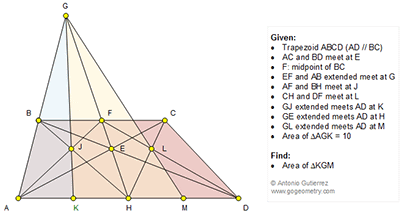## Sunday, February 28, 2016

### Geometry Problem 1192: Trapezoid, Triangle, Diagonal, Midpoint, Area, Parallel

Geometry Problem. Post your solution in the comment box below.
Level: Mathematics Education, High School, Honors Geometry, College.

Click the figure below to view more details of problem 1192.#### 1 comment:

1.From similar triangles, BF/HD = FE / EH = CF/AH and since BF = CF it follows that AH = HD.

Also from similar triangles BF/AH = GF/GH = FC/HD since F and H are respectively the midpoints of BC and AD.

This (and since < GFC = < HDG) implies that triangles GFC and GHD are similar and so < FCG = < HDG thus implying that D,C,G are collinear points.

Thus we have proved that in trapezoid ABCD, GE bisects the parallel sides.

This is also true for trapezoids ABFH and CDFH.

Hence AK=KH=HM=MD

Hence S(KGM) = 2 X S(AGK) = 20

Sumith Peiris
Moratuwa
Sri Lanka